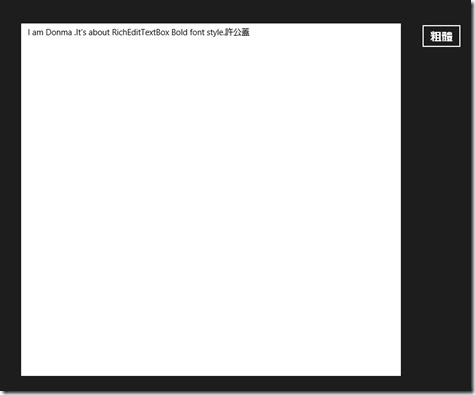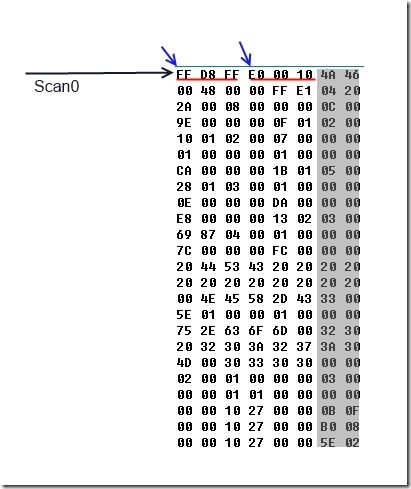2012-12-26

## [C#][Win8] RichEditBox 選取文字改粗體

RicjTextEdior 在  Windows 8 App 開發中，跟以前有一點差異，這邊做一個很簡單的範例.

http://msdn.microsoft.com/zh-TW/library/windows/apps/windows.ui.text.itextcharacterformat
http://msdn.microsoft.com/en-us/library/windows/apps/BR2275482012-12-24

## [C#] XML format convert to JSON

2012-12-15

`<?xml version="1.0" encoding="UTF-8" standalone="yes"?>`
`<Root xmlns:xsi="http://www.w3.org/2001/XMLSchema-instance">`
`    `
`  <Row>`
`        <use>0</use>`
`        <page>1</page>`
`        <pic_file_name>a.jpg</pic_file_name>`
`        <product_name>A商品</product_name>`
`        <url>http://www.msdn.com</url>`
`    </Row>`
`    <Row>`
`        <use>1</use>`
`        <page>1</page>`
`        <pic_file_name>b.jpg</pic_file_name>`
`        <product_name>B商品</product_name>`
`        <url>http://www.msdn.com</url>`
`    </Row>`
`    <Row>`
`        <use>2</use>`
`        <page>1</page>`
`        <pic_file_name>c.jpg</pic_file_name>`
`        <product_name>C商品</product_name>`
`        <url>http://www.msdn.com</url>`
`    </Row>`
`    <Row>`
`        <use>3</use>`
`        <page>2</page>`
`        <pic_file_name>d.jpg</pic_file_name>`
`        <product_name>D商品</product_name>`
`        <url>http://www.msdn.com</url>`
`    </Row>`
`    <Row>`
`        <use>4</use>`
`        <page>2</page>`
`        <pic_file_name>e.jpg</pic_file_name>`
`        <product_name>E商品</product_name>`
`        <url>http://www.msdn.com</url>`
`    </Row>`
`    `
`</Root>`

2012-12-14

## [C#] 濾鏡筆記 - 負片效果(Invert)

2012-12-13

Invert 英文叫做顛倒.. 原理很簡單也就是 將 255- 原本的值..

C# Code:

`public System.Drawing.Bitmap ConvertToInvert(System.Drawing.Image img) {    // 讀入欲轉換的圖片並轉成為 Bitmap    System.Drawing.Bitmap bitmap = new System.Drawing.Bitmap(img);    for (int y = 0; y < bitmap.Height; y++)    {        for (int x = 0; x < bitmap.Width; x++)        {            // 取得每一個 pixel            var pixel = bitmap.GetPixel(x, y);            // 負片效果 將其反轉            System.Drawing.Color newColor = System.Drawing.Color.FromArgb(pixel.A, 255 - pixel.R, 255 - pixel.G, 255 - pixel.B);            bitmap.SetPixel(x, y, newColor);        }    }    // 結果    return bitmap;}`

2012-12-12

2012-12-11

## [C#] 透過WebClient Post Data

2012-12-10

Server 透過 WebClient 使用POST 傳送資料…

`Response.Write(Request.Form["id"]+","+Request.Form["age"]+".");`

`string targetAddress = "http://localhost:1550/risiv.aspx";`
`string parameters = "id=許公蓋&age=12";`
`wc.Encoding = Encoding.UTF8;`
`wc.Headers[HttpRequestHeader.ContentType] = "application/x-www-form-urlencoded";`
`string result = wc.UploadString(targetAddress, parameters);`
` `
` `
`Response.Write(result);`

parameter為參數值

## [C#] 濾鏡筆記 – LockBits影像處理 + 浮雕效果

2012-12-09## [C#] 濾鏡筆記 – 高對比

2012-12-08

(((( 顏色色值 / 255.0) - 0.5) * 影響度 0~4 之間 ) + 0.5) * 255.0

## [C#] 濾鏡筆記 – 偵測人的皮膚

2012-12-07

`public static bool IsSkinColor(byte r, byte g, byte b)`
`{`
` `
`   // 公式 1 : http://www.codeproject.com/Articles/38176/Image-Processing-Skin-Detection-Some-Filters-and-E`
` `
`   //return ( (r > 95) && (g > 40) && (b < 20)) && ( (Math.Max(Math.Max(r, g), b)) - (Math.Min(Math.Min(r, g), b)) > 15) &&`
`   //       (Math.Abs(r - g) > 15) && (r > g) && (r > b) ||`
`   //       ( (r > 220) && (g > 210) && (b > 170) && (Math.Abs(r - g) == 15) && (r > b) && (g > b));`
` `
` `
`   // 公式 2 :http://www.codeproject.com/Articles/38176/Image-Processing-Skin-Detection-Some-Filters-and-E`
`   //if (r == 0 || b == 0 || g == 0) return false; `
` `
`   //return ((r/g) > 1.185) && (((r*b)/((r + g + b)*(r + g + b))) > 0.107) &&`
`   //       (((r*g)/((r + g + b)*(r + g + b))) > 0.112);`
` `
` `
` `
`   // 公式 3 :http://www.codeproject.com/Articles/8127/Skin-Recognition-in-C`
`   //double I = (Math.Log(r) + Math.Log(b) + Math.Log(g)) / 3;`
`   //double Rg = Math.Log(r) - Math.Log(g);`
`   //double By = Math.Log(b) - (Math.Log(g) + Math.Log(r)) / 2;`
`   //double hue = Math.Atan2(Rg, By) * (180 / Math.PI);`
` `
` `
`   //if (I <= 5 && (hue >= 4 && hue <= 255))`
`   //{`
`   //    return true;`
`   //}`
`   //else`
`   //{`
`   //    return false;`
`   //}`
` `
` `
`   // 公式四 : http://w3.ualg.pt/~ftomaz/download/dicta2003.pdf`
`   var Cb = (0.148 * r) - (0.291 * g) + (0.439 * b) + 128;`
`   var Cr = (0.439 * r) - (0.368 * g) - (0.071 * b) + 128;`
` `
`   if ((r > 95 && g > 40 && b > 20 && (Math.Max(Math.Max(r, g),`
`   b) - Math.Min(Math.Min(r, g), b) > 15) && (r - g) > 15 &&`
`   r > g && r > b) &&`
`   (Cr > 140 && Cr < 162 || Cb > 105 && Cb < 130))`
`   {`
`       return true; `
`   }`
`   else`
`   {`
`       return false; `
`   } `
` `
` `
`}`

## [C#] 濾鏡筆記 – 調整Gamma數值

2012-12-06

Gamme 是因應人類視覺特性而生的一種色彩處理方式。人類視覺特性之一 ，在微光的敏銳度大於強光中的敏銳度

## [C#] 濾鏡筆記 – 銳利化

2012-12-05

http://stackoverflow.com/questions/903632/sharpen-on-a-bitmap-using-c-sharp

http://blog.csdn.net/jiangxinyu/article/details/6222322

`// 建立3x3 矩陣 拉普拉斯`
`//  -1  -1  -1    (0,0) | (1,0) | (2,0) `
`//  -1  +9  -1    (0,1) | (1,1) | (2,1)`
`//  -1  +1  -1    (0,2) | (1,2) | (2,2)`

## [C#] 濾鏡筆記 – 漣漪效果

2012-12-04

http://www.codeproject.com/Articles/2812/A-C-water-effect-picture-control

http://www.aforgenet.com/framework/docs/html/6f6ba0e1-cc41-0277-c856-598089b4ed02.htm

http://www.codeproject.com/Articles/3419/Image-Processing-for-Dummies-with-C-and-GDI-Part-5

## [C#] 濾鏡筆記 – 色調濾鏡

2012-12-03

`/// <summary>`
`/// 純紅濾鏡`
`/// </summary>`
`/// <param name="bitmap">Bitmap</param>`
`/// <param name="threshold">門檻值 -255~255</param>`
`/// <returns></returns>`
`public System.Drawing.Bitmap AdjustToRed(System.Drawing.Bitmap bitmap, int threshold)`
`{`
`    for (int y = 0; y < bitmap.Height; y++)`
`    {`
`        for (int x = 0; x < bitmap.Width; x++)`
`        {`
`            // 取得每一個 pixel`
`            var pixel = bitmap.GetPixel(x, y);`
`            var pR = pixel.R + threshold;`
`            pR = Math.Max(pR, 0);`
`            pR = Math.Min(255, pR);`
`            // 將改過的 RGB 寫回`
`            // 只寫入紅色的值 , G B 都放零`
`            System.Drawing.Color newColor = System.Drawing.Color.FromArgb(pixel.A, pR, 0, 0);`
`            bitmap.SetPixel(x, y, newColor);`
`        }`
`    }`
`    // 回傳結果`
`    return bitmap;`
`}`

2012-12-02

2012-12-01